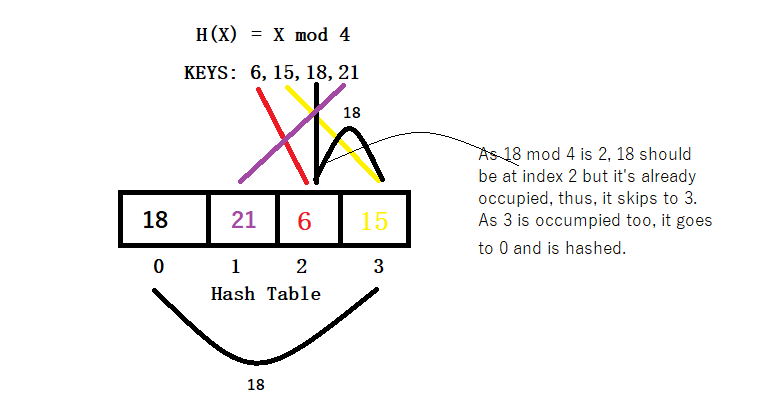# Linear Probing

Posted: 20 Dec, 2020
Difficulty: Easy

## PROBLEM STATEMENT

#### You can refer to the example given below:#### Note:

``````1. Consider ‘0’ based indexing.
``````
##### Input format:
``````The first line of input contains an integer ‘T’ denoting the number of test cases.

The first line of each test case contains a positive integer ‘N’ representing the size of array KEYS.

The second line of each test case contains ‘N’ space-separated non-negative integers representing elements of the array KEYS.
``````
##### Output format :
``````For each test case, return an array 'HASH_TABLE’ of size ‘N’ where HASH_TABLE[ i ] is the element from the given array KEYS which is mapped to the index i.
``````
##### Note:
``````You do not need to print anything, it has already been taken care of. Just implement the given function.
``````
##### Constraints:
``````1 <= T <= 10
1 <= N <= 500
0 <= KEYS[ i ] <= 10^9

Time limit: 1 sec
``````Approach 1

The approach is pretty simple. We just need to find the mapping index and if it is occupied, we need to search for the next available index.

The algorithm for the same is as follows:

• Create an array HASH_TABLE of size N.
• Initialise every value of HASH_TABLE as -1.
• Loop for each KEY:
• Initialize HASH = KEY % N
• If HASH_TABLE[ HASH ] is already occupied, we perform:
• while(HASH_TABLE[ HASH ] != -1):
• HASH = ( HASH + 1 ) % N, iterating for valid hash index.
• Update HASH_TABLE[ HASH ] with KEY.
• Return HASH_TABLE

Let’s take an example of keys = { 91, 28, 46 }.

• Initialise HASH_TABLE = { -1, -1, -1 }
• For 91, H(91) = 91 mod 3 i.e. 1.
• As index 1 is free, HASH_TABLE becomes { -1, 91, -1 }.

• For 28, H(28) = 28 mod 3 i.e. 1.
• As index 1 is already occupied, we will move to while loop:
• We use index 2, which is free, HASH_TABLE becomes { -1, 91, 28 }

• For 46, H(46) = 46 mod 3 i.e. 1.
• As index 1 is occupied, we move to while loop:
• HASH = (1 + 1) mod 3 i.e. 2. As index 2 is occupied, loop continues.
• HASH = (2 + 1) mod 3 i.e. 0. Index 0 is free, thus, we will use index 0.

The final HASH_TABLE becomes { 46, 91, 28 }